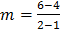### Sample Problem

A line goes through the points (1, 4) and (2, 6). Write the equation of this line in slope-intercept form.

y = x +

#### Solution

A linear equation of a slope-intercept form y = mx + b , m is the slope and b is the y-intercept.

We know two points on the line, so we can calculate the slope.= 2.

Next, we can find the y-intercept by plugging in one point (1,4) we know and the m value, then solve for b. 4 = 2(1) + b; b = 2.

The equation of this line is therefore y = 2x + 2.